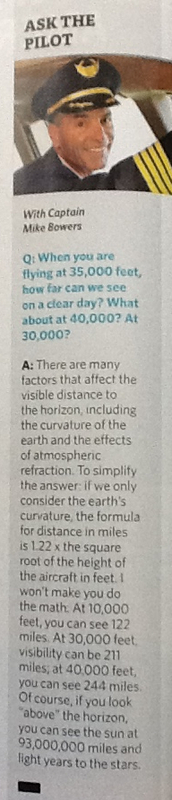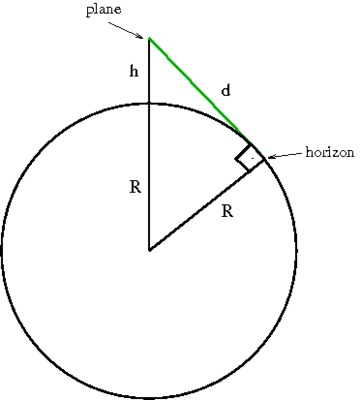##### Engage your students with effective distance learning resources. ACCESS RESOURCES>>

Alignments to Content Standards: G-SRT.C.8

In the July 2013 issue of United Airlines' Hemisphere Magazine the following article appeared:1. Write down an equation that describes Captain Bowers' method.
2. Create your own mathematical model to find the distance to the horizon from a plane.
3. Compare the two models. What simplifying assumptions did Captain Bowers make to create his model? Are these assumptions justified?

## IM Commentary

The article from an airline magazine gives an answer to a mathematical question. The pilot used a mathematical model to simplify the computation of finding the distance to the horizon at different airplane heights. The underlying mathematical model is based on right triangles and lines of tangency to a circle. After drawing the right diagram, the problem can be solved with a very straight forward application of the Pythagorean Theorem. It is the same model that is used in

Captain Bowers could have given a method that would lead to more accurate answers but it would have been much more complicated. Given the purpose of the computation and the level of accuracy, or inaccuracy, of the numbers involved (the radius of the earth), his answer is a perfect example for appropriate simplification in mathematical modeling.

More questions to the pilot can be found at https://hub.united.com/en-us/news/company-operations/pages/ask-the-united-pilot-july-2013.aspx

This task can be used as a classroom activity. There is a lot of opportunity to discuss the process of mathematical modeling. It serves to illustrate MP 4 - Model with Mathematics, not just by engaging in the practice, but also by investigating what this practice entails. Since students are evaluating a given model, they are also asked to critique the reasoning of others, part of MP 3.

## Solution

1. Captain Bowers said that the "distance in miles is 1.22 $\times$ the square root of the height of the aircraft in feet". As an equation this is $$d=1.22 \sqrt{h},$$ where $d$ is the distance from the plane to the horizon in miles and $h$ is the height of the aircraft, in feet.
2. Captain Bowers is only considering the curvature of the earth as the main factor for his computation. We can draw a diagram that shows the geometry involved in the problem:In the diagram, the circle is the cross section of the earth, the plane is $h$ ft above the earth and the radius of the earth is given by $R$. We are trying to find $d,$ the distance from the plane to the horizon in miles.

Note that the green line segment of length $d$ is tangent to the circle, and therefore it is perpendicular to the radius of the circle. Using the Pythagorean Theorem we have: $$R^2+d^2 = (R+h)^2.$$ Solving for $d$ we have:

\begin{aligned} (R+h)^2&=R^2+d^2\\ R^2+2hR+h^2 &= R^2+d^2\\ d^2 &= 2hR+h^2\\ d &= \sqrt{2hR+h^2}.\\ \end{aligned}

The radius of the earth is about 3960 miles, therefore, $R=3960\text{ miles}$. The height $h$ in Captain Bowers calculation is given in feet but the distance is given in miles, so we have to convert units. We can do this by dividing the height by 5280 since 1 mile = 5280 feet. Therefore we have:

\begin{aligned} d &= \sqrt{2\cdot 3960\cdot \frac{h}{5280}+\left(\frac{h}{5280}\right)^2}\\ &=\sqrt{1.5 h+\left(\frac{h}{5280}\right)^2} \end{aligned}
We can't simplify this equation any further, so this will be our model.
3. We now compare the two methods. Both methods make the same assumption that the earth is a perfect sphere, with a radius of close to $3960$ miles. They also both assume that there are no obstructions in the line of sight such as mountain ranges or haze in the air. However, Captain Bowers' equation is considerably simpler than our equation. He must be making additional assumptions.

Captain Bowers' method: $d=1.22 \sqrt{h}$

Our method: $d=\sqrt{1.5 h+\left(\frac{h}{5280}\right)^2}$

Note that $1.22 \approx \sqrt{1.5}$. Comparing our equation with Captain Bowers', it looks like he omitted the last term under the square root sign, $\left(\frac{h}{5280}\right)^2$.
4. To see if this simplification is justified, we can make a table of values to compare our model with Captain Bowers' model.
height $h$ feet $\sqrt{1.5 h+\left(\frac{h}{5280}\right)^2}$ miles $1.22 \sqrt{h}$ miles
10 3.87 3.86
100 12.2 12.2
1000 38.7 38.6
10,000 122 122
30,000 212 211
40,000 245 244

It seems that omitting $\left(\frac{h}{5280}\right)^2$ from the calculation has little effect, the distances using both methods are very close to each other. Looking back at the equation $$d=\sqrt{1.5 h+\left(\frac{h}{5280}\right)^2}$$ we can explain why this is the case by comparing the relative sizes of the two terms. $1.5h$ is much larger than $\left(\frac{h}{5280}\right)^2$. For example, for $h=10,\!000$, $1.5h=15,\!000$ and $\left(\frac{h}{5280}\right)^2\approx 3.59.$ The two numbers differ by 4 orders of magnitude. Therefore, omitting the relatively small number that gets tagged on, has little effect on the final answer.

Captain Bowers' simplification is not only justified, it is an excellent idea. It simplifies the computation considerably without really changing the accuracy of the answer.